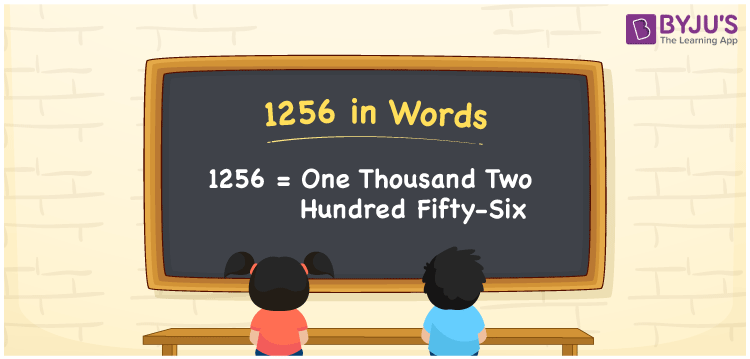# 1256 in Words

We can write 1256 in words as One thousand two hundred fifty-six. If you paid Rs. 1256 as a house tax, then you can say, “I paid One thousand two hundred fifty-six rupees for house tax”. Therefore, we can represent the cardinal number 1256 in word form as “One thousand two hundred fifty-six”, which is also called the number name for 1256. In this article, you will learn how to convert 1256 into words with the help of a place value chart.

 1256 in words One thousand two hundred fifty-six One thousand two hundred fifty-six in Numbers 1256

## 1256 in English Words

We generally write numbers in words using the English alphabet. Thus, we spell 1256 in words as “One thousand two hundred fifty-six”.## How to Write 1256 in Words?

1256 is a four-digit number so, let’s create a place value chart with four columns, as given below.

 Thousands Hundreds Tens Ones 1 2 5 6

Here, ones = 6, tens = 5, hundreds = 2, and thousands = 1.

Thus, 1 × Thousand + 2 × Hundred + 5 × Ten + 6 × One

= 1 × 1000 + 2 × 100 + 5 × 10 + 6 × 1

= 1000 + 200 + 50 + 6

= One thousand + Two hundred + Fifty + Six

= One thousand two hundred fifty-six

Therefore, 1256 in words = One thousand two hundred fifty-six.

Learn in detail about place value here.

As we know, 1256 is a natural number that precedes 1257 and succeeds 1255.

1256 in words – One thousand two hundred fifty-six

Is 1256 an odd number? – No

Is 1256 an even number? – Yes

Is 1256 a prime number? – No

Is 1256 a composite number? – Yes

Is 1256 a perfect square number? – No

Is 1256 a perfect cube number? – No

## Frequently Asked Questions on 1256 in Words

Q1

### What is the word form of 1256?

One thousand two hundred fifty-six is the word form of 1256, i.e., 1256 = One thousand two hundred fifty-six.
Q2

### How to write Rs. 1256 in words on a cheque?

On a cheque, we generally write Rs. 1256 in words as “One thousand two hundred fifty-six rupees only”.
Q3

### How to read 1256 in words?

The spelling of 1256 in English words is One thousand two hundred fifty-six. Therefore, we can read the number 1256 as One thousand two hundred fifty-six.